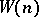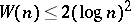# Difference between revisions of "Pseudo-prime"

Traditionally, a composite natural number $n$ is called a pseudo-prime if $2^{n-1} \equiv 1$ modulo $n$, for it has long been known that primes have this property. (The term is apparently due to D.H. Lehmer.) There are infinitely many such $n$, the first five being $$341,\,561,\,645,\,1105,\,1387\ .$$

More recently, the concept has been extended to include any composite number that acts like a prime in some realization of a probabilistic primality test. That is, it satisfies some easily computable necessary, but not sufficient, condition for primality. Pseudo-primes in this larger sense include:

1) ordinary base-$b$ pseudo-primes, satisfying $b^{n-1}\equiv1$ modulo $n$;

2) Euler base-$b$ pseudo-primes, whose Jacobi symbol with $b$ satisfies $$b^{(n-1)/2} \equiv\left({\frac{b}{n}}\right) = \pm 1$$

3) strong base-$b$ pseudo-primes, for which the sequence $b^{s.2^i}$ modulo $n$ for $i=0,\ldots, r$ is either always $1$, or contains $-1$. (Here $n-1 = 2^r.s$ with $s$ odd.)

For each, the implications 3)2)1) hold. A numberthat is an ordinary base-pseudo-prime for allprime tois called a Carmichael number. Analogous numbers for the other two categories do not exist.

For a thorough empirical study of pseudo-primes, see [a4]. Lists of pseudo-primes to various small bases can be found in [a6].

The concept of a pseudo-prime has been generalized to include primality tests based on finite fields and elliptic curves (cf. also Finite field; Elliptic curve). For reviews of this work, see [a3], [a5].

The complementary concept is also of interest. The baseis called a (Fermat) witness forifis composite but not a base-pseudo-prime. Euler and strong witnesses are similarly defined. If, the smallest strong witness for, grows sufficiently slowly, there is a polynomial-time algorithm for primality. It is known thatis not bounded [a2], but if an extended version of the Riemann hypothesis (cf. Riemann hypotheses) holds, then[a1].

How to Cite This Entry:
Pseudo-prime. Encyclopedia of Mathematics. URL: http://encyclopediaofmath.org/index.php?title=Pseudo-prime&oldid=16100
This article was adapted from an original article by E. Bach (originator), which appeared in Encyclopedia of Mathematics - ISBN 1402006098. See original article